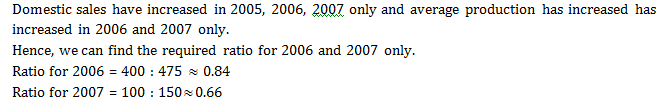Direction for question 1– 6: Answer the questions based on the following graphs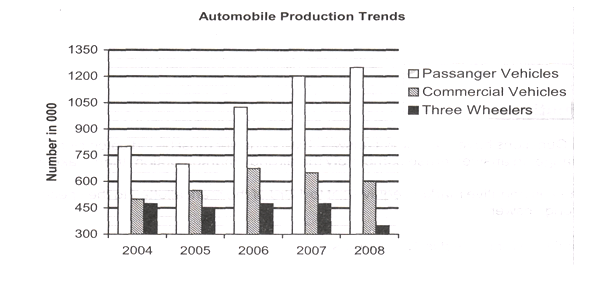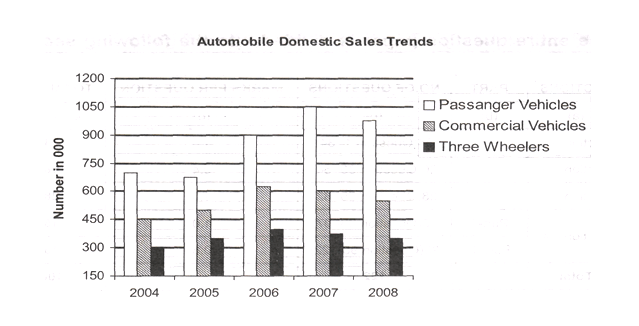Question 1.  Which of the following year exhibited highest percentage decrease over the preceding year in the automobile production?
A. 2005
B.2006
C.2007
D.2008

Question 2.  Assuming whatever that is not sold domestically was exported, then which year has registered highest growth inexports of automobiles?
A.2005
B.2006
C.2007
D.2008

Question 3. If the ratio of the domestic sale price of a commercial vehicle, a passenger vehicle, and a three wheeler is 5:3:2 then what percent of earnings (approximately) is contributed by commercial vehicle segment to the overall earnings from domestic sales during the period 2004-2008?
A. 45%
B. 43%
C. 11%
D. 27%

Question 4.  For which year were the domestic sales of automobiles closest to the average (2004-2008) domestic sales of automobiles?
A. 2005
B.2006
C. 2007
D.2008

Question 5.  Which of the following years exhibited highest percentage increase over the preceding year in the
automobile sales?
A. 2005
B.2006
C. 2007
D. 2008

Question 6. The ratio between absolute increase in domestic sales over preceding year and absolute increase in production over the preceding year is highest during which year?
A. 2005
B.2006
C. 2007
D.2008

The automobile production in 2004, 2005, 2006, 2007 and 2008 are 1775, 1700, 2175, 2325 and 2200 respectively.

The production shows decrease only in the year 2005 and 2008

The percentage decrease in 2005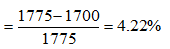Percentage decrease in 2008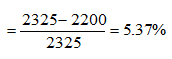Hence, 2008 exhibited highest percentage decrease.

In year 2004, exports of automobiles = production – sales = 1775 – 1450 = 325

In year 2005, export of automobiles = 1700 – 1525 = 17

In year 2006, export of automobiles = 2175 – 1925 = 250

In year 2007, export of automobiles = 2325 – 2025 = 300

In year 2008, export of automobiles = 2200 – 1875 = 325

So highest growth in export is in year 2006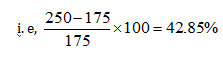Let 5x, 3x, and 2x be the prices of a commercial vehicle, passenger vehicle and 3 wheeler respectively.

In the period 2004 – 08, earnings from domestic sales of :

Commercial vehicle = 2725 × 5x = 13625x

Passenger vehicle = 4300 × 3x = 12900x

3 wheeler = 1775 × 2x = 3550x

∴ Total sales = 30075x

∴ Required percentage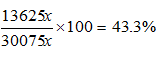Average domestic sales = 1760

Closest year with sales is 2008 i.e., 1875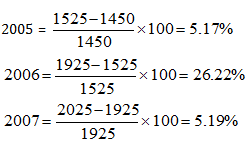Answer 6. (B)